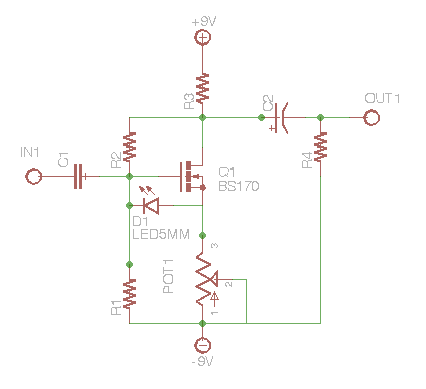# MosFET Gain

Here’s a typical MosFET boost. The gain is roughly determined by the ratio of Drain and Source resistors. Divide the Drain resistor (Rd) by the Source resistor (Rs) to find the gain. For example if Rd = 5k and Rs = 5k the gain is 1.
Use a Pot for Rs to set variable gain. Imagine POT1 as a 5k pot. Notice how the gain changes exponentially.

• 5k/5k = 1
• 5k/4k = 1.25
• 5k/3k = 1.67
• 5k/2k = 2.5
• 5k/1k = 5
• 5k/.5k = 10

Use a Reverse Log pot to get the linear feel for the gain control. (Thanks to Nelson on the forum for this tip.)Posted

in

by

Tags: